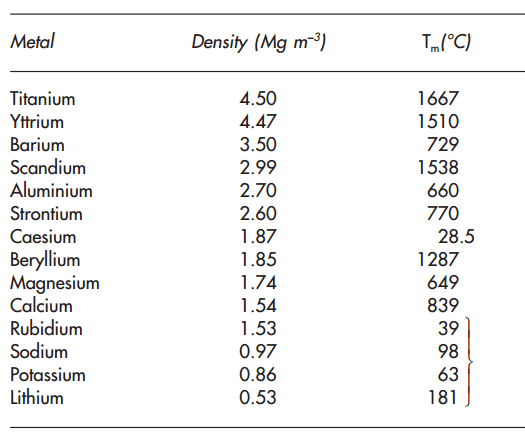# Density Formula: Meaning, Definition, Solved Examples

Density is a basic and fundamental concept in physics and engineering. Furthermore, it has a strong relationship with the mass of an object. Moreover, this measurement is essential in determining whether something would float or not on a fluid’s surface. Density is certainly one of the most important details you can know about an object. Learn the density formula here.

## What is Density?

Density refers to the measurement of the amount of mass of a substance per unit of volume. This measurement of a pure substance has the same value as its mass concentration. Densities vary with different materials or substances. Moreover, this particular measurement of a material can be relevant to purity, buoyancy, and packaging.

One can simplify this measurement’s comparisons across the different systems of units. The replacement of this measurement with a concept is known as relative density can sometimes take place. Relative densities refer to the ratio of a substance’s density to a standard substance or material, usually water.The densities of materials show variation with pressure and temperature. This variety is comparatively small for solids and liquids but significantly greater when it comes to gases. When the pressure on an object increases, then consequently the volume decreases. This ultimately causes an increase in this particular measurement of the object.

Also, increasing a substance’s temperature results in a decrease in this measurement. This is due to the increase in volume. Heating the bottom of a liquid in most cases results in a decrease in this measurement of such heated liquid.

### Density Formula

Densities refer to the measure of relative compactness. The definition of this measure can be expressed as mass, m, in a particular volume, v. Most noteworthy, the symbol ρ is mostly made use of for expressing this measurement. Furthermore, this symbol happens to be the lower case Greek letter rho. Some individuals also make use of the letter D for expressing this measurement.

### Solved Example on Density Formula

Q1. Find out the density of a cube of sugar which weighs 12 grams and measures 2 cm on a side?

Answer: This question can be completed in two steps

Step 1: Derive the mass and volume of this sugar cube

Mass = 12 grams

Volume = 2 cm sides

The volume of a cube = (length of side)3

Volume of cube = (length of side)3

Volume = (2)3

Volume = 8 cm3

Step 2: Putting the variables into the formula

Density = mass/volume = 12 grams/8 cm3

So, it comes out to be = 1.5 grams/cm3

Hence, this sugar cube has a density of 1.5 grams/cm3.

Q2. A particular solution of salt water contains 20 grams of salt and 200 grams of water. Find out the density of the salt water? (note = density of water is 1 g/mL)

Answer: Step 1: Derive the mass and volume of the salt water.

Mass of salt = 20 grams

Density of water = mass of water/volume of water

Mass of water = 1 g/mL multiplied by 200 mL

Therefore, the mass of water = 200 grams

Now, one can find out the mass of the saltwater

Mass of salt water = mass salt + mass of water

Mass of salt water = 20 g + 200 g

So, mass of salt water = 220 g

Also, the volume of salt water is 200 mL.

Step 2: Putting these values into the formula

Density = mass/volume = 220 g/200 mL

So, it comes out to be = 1.1 g/mL

Hence, this salt water certainly has a density of 1.1 g/mL.